#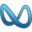RandomFields¶

Fopt Known Xopt Known Difficulty
No No Hard

The approach used in the RandomFields test suite is described in https://www.researchgate.net/publication/301940420_Global_optimization_test_problems_based_on_random_field_composition . It generates benchmark functions by “smoothing” one or more multidimensional discrete random fields and composing them, and it is composed by three different steps:

1. A basic multidimensional discrete random field generator capable to produce parameterized fields with higher order interactions.
2. A “smoothing” method to obtain continuous and differentiable fields, by means of weighting functions.
3. A composition technique to create structured fields by combining different types of basic random fields.

No source code is given, but the implementation is fairly straightforward from the article itself.

##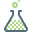Methodology¶

For the Multidimensional Discrete Random Field (MDRF) generator, the generator function (referred to as operator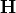), takes a multidimensional vector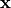of floating point values from the unit hypercube domain as an input, and maps it to a value from a given set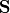with a discrete probability distribution and a computational type (e.g. binary integer or float) of choice: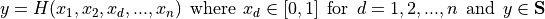To explain the concept of the implementation, a related discretized version of this idea can be defined as: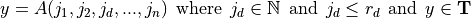Operator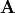can be interpreted as high-dimensional random “array” A with indices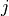of which each index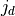is bounded by the maximum array size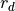for dimension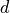. In the second expression,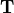is a finite set of successive integers pointing to the distinct elements of the set.

The concept chosen for the MDRF generator algorithm is to compute and reproduce the pseudo-random values of the high-dimensional arrays “on the fly” instead of storing a potentially huge passive map in the computer memory. Another alternative interpretation would be to consider operator :math:A as a pseudorandom number generator with a high-dimensional vector as its generating seed.

Using a multiplicative weighting function as expressed in equation below these discrete random fields can be transformed to “smooth” differentiable continuous random fields: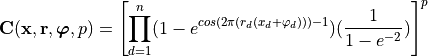Where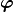denotes the vector of the (phase) shift of the discrete field and the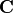operator such that also fields with non-zero values along the domain boundaries can be constructed (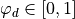). With parameter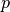the shape of the function can be adjusted.

The application of the “bare bones” discrete random fields generated by the algorithm above is of little practical interest because of the primitive problem structure. Compositions of such fields of different and heterogeneous resolutions, dimensions and codomain distributions can provide test functions with interesting problem structures. Continuous fields with different spatial resolution can be created, and compositions can be made by for example multiplication or by weighted addition such as for example: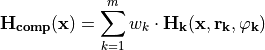where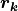and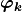(both in bold) denote the vectors with the array size and shifts for each dimension of composition fields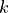.

To cut the story short, my approach in generating the test functions for the RandomFields benchmarks hs been:

1. The dimensionality of the problem varies between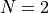and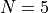2. The number of compositions functions can be 1, 2, 4, 6, 8 or 10
3. The exponentcan be 0.25, 0.5, 1, 2, or 3
4. The shift can be any of 0, 0.2, 0.4 or 0.6

In this way, I have generated 120 test functions for each dimension, for a total of 480 benchmarks.

One of the main differences between the RandomFields benchmark and most of the other test suites is that neither the global optimum position nor the actual minimum value of the objective function is known. So, in order to evaluate the solvers performances in a similar way as done previously, I have elected to follow this approach, for each of the 480 functions in the RandomFields test suite:

1. Generate a brute-force grid of N points between 0 and 1 (the actual bounds). This grid is composed by at least 1,000,000 points (depending on the problem dimensions).
2. Evaluate the objective function at each of these points.
3. After identifying the most promising 1,000 points in the grid (i.e., points for which the function value is lowest), start 3 different local optimization algorithms from each of these points. The algorithms selected for this exercise were a mix of derivative-free and gradient-based approaches (L-BFGS-B, Subplex, Power methods).
4. Out of all these minimizations, the lowest function value obtained becomes the putative “global minimum” for the current benchmark function.

A few examples of 2D benchmark functions created with the RandomFields generator can be seen in Figure 10.1.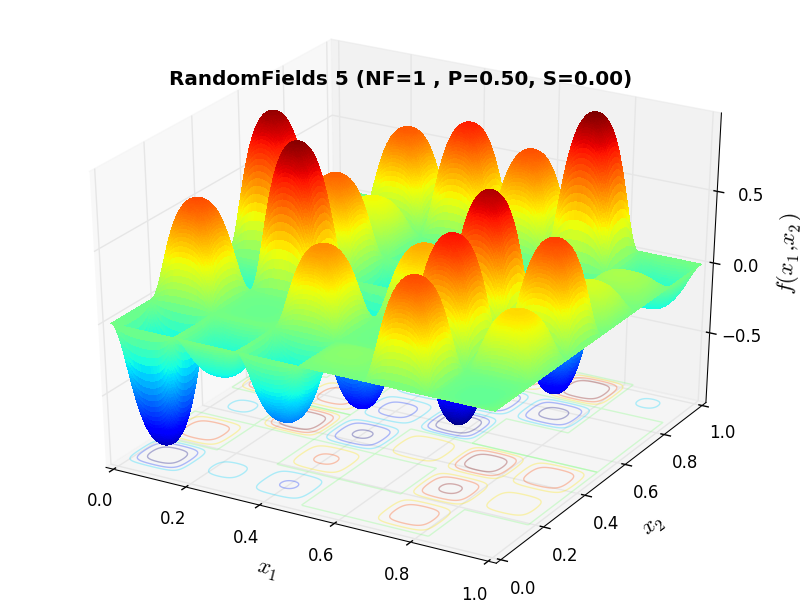RandomFields Function 5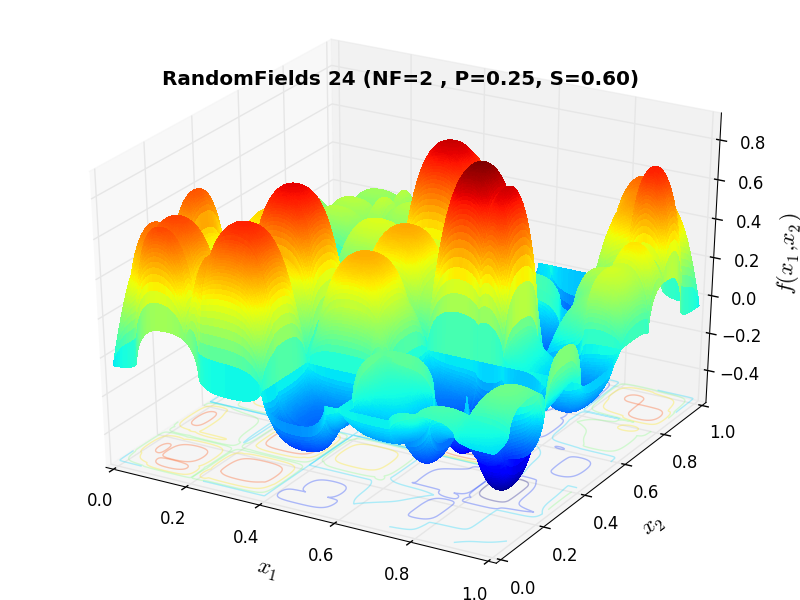RandomFields Function 24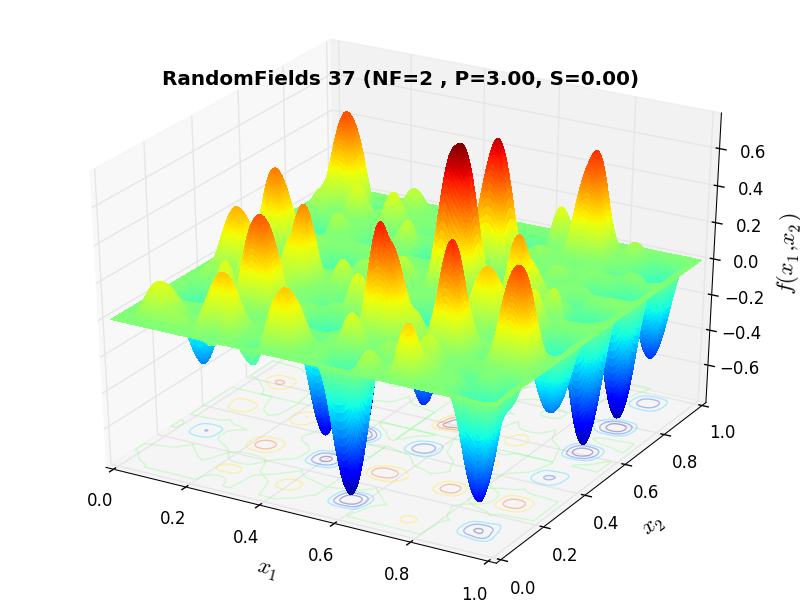RandomFields Function 37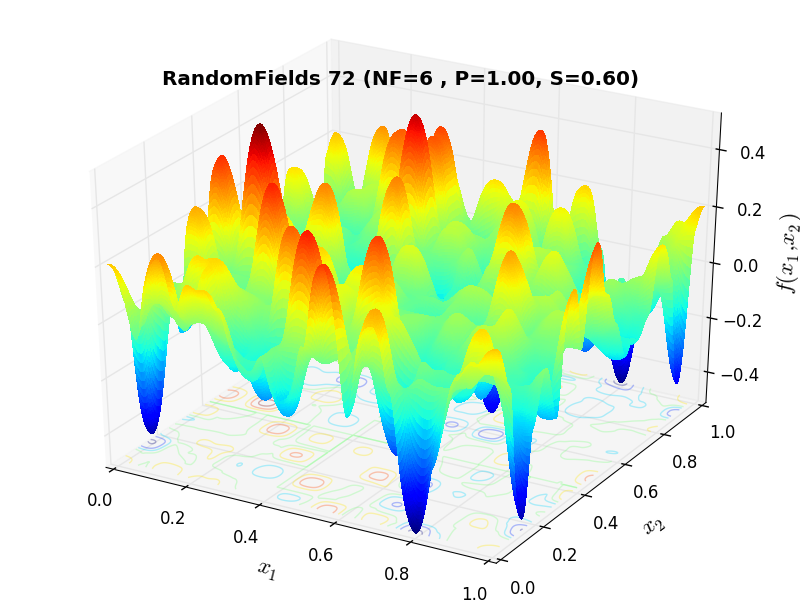RandomFields Function 72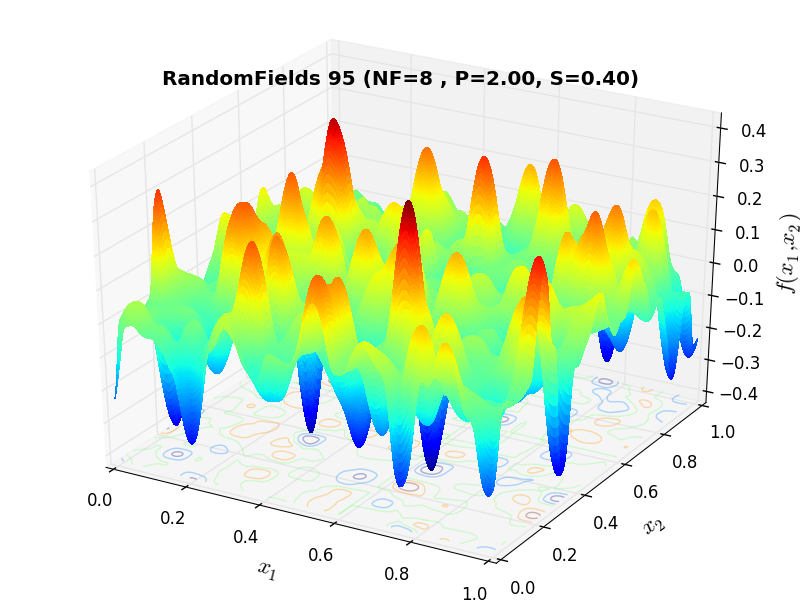RandomFields Function 95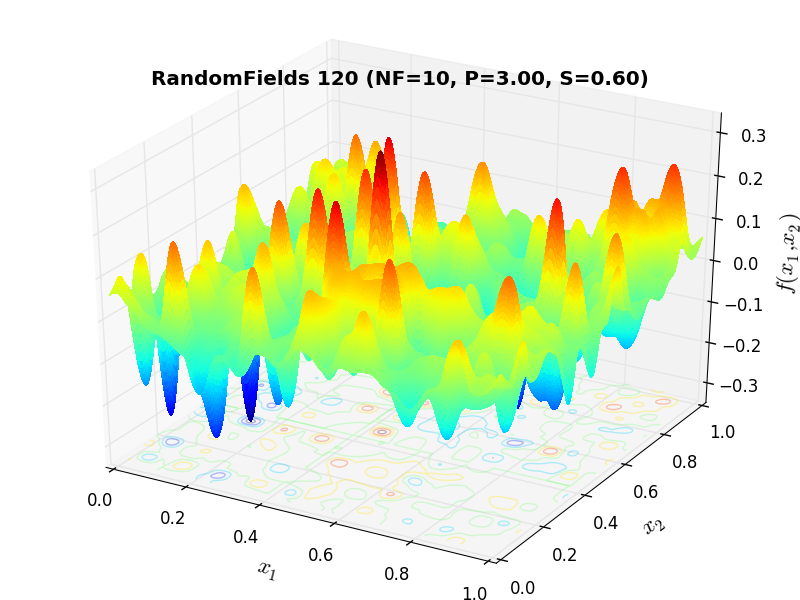RandomFields Function 120

##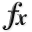General Solvers Performances¶

Table 10.1 below shows the overall success of all Global Optimization algorithms, considering every benchmark function, for a maximum allowable budget of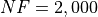.

The RandomFields benchmark suite is a relatively hard test suite: the best solver foris DIRECT, with a success rate of 34.4%, but DualAnnealing and SHGO achive pretty much the same results, closely followed by MCS.

Note

The reported number of functions evaluations refers to successful optimizations only.

Table 10.1: Solvers performances on the RandomFields benchmark suite at NF = 2,000
Optimization Method Overall Success (%) Functions Evaluations
AMPGO 10.62% 880
BasinHopping 19.38% 678
BiteOpt 24.79% 598
CMA-ES 9.17% 978
CRS2 13.12% 691
DE 19.79% 1,091
DIRECT 34.38% 435
DualAnnealing 34.17% 510
LeapFrog 8.33% 193
MCS 29.38% 614
PSWARM 14.37% 1,648
SCE 17.08% 786
SHGO 33.96% 513

These results are also depicted in Figure 10.2, which shows that DIRECT, DualAnnealing and SHGO are the better-performing optimization algorithms, followed by MCS.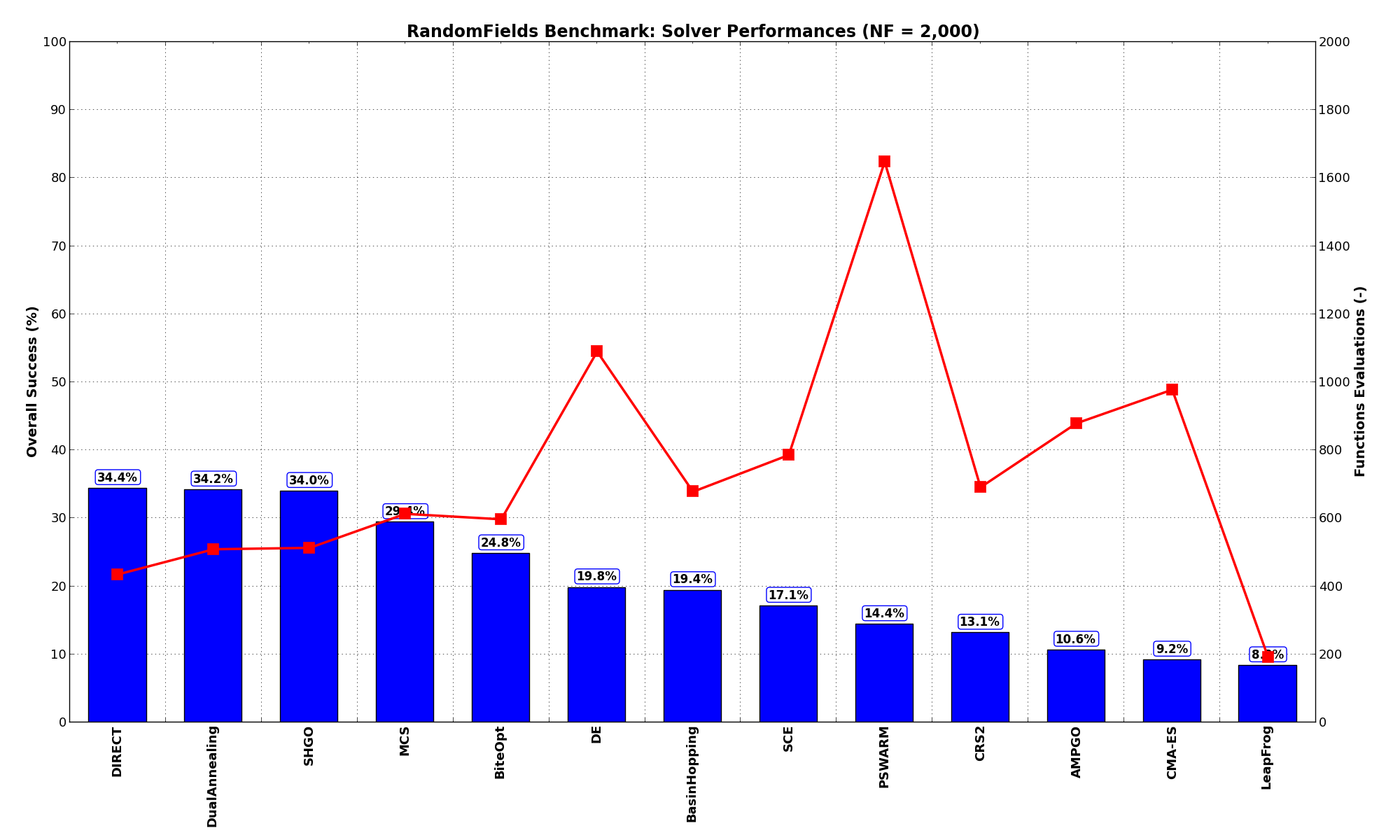Figure 10.2: Optimization algorithms performances on the RandomFields test suite atPushing the available budget to a very generous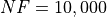, the results show SHGO taking the lead, with MCS following by a large margin. The results are also shown visually in Figure 10.3.

Table 10.2: Solvers performances on the RandomFields benchmark suite at NF = 10,000
Optimization Method Overall Success (%) Functions Evaluations
AMPGO 59.17% 2,319
BasinHopping 88.33% 1,312
BiteOpt 38.96% 956
CMA-ES 52.29% 1,665
CRS2 37.08% 1,032
DE 47.08% 1,820
DIRECT 68.75% 962
DualAnnealing 57.29% 791
LeapFrog 23.75% 270
MCS 87.08% 1,614
PSWARM 47.08% 2,110
SCE 43.12% 806
SHGO 80.00% 1,206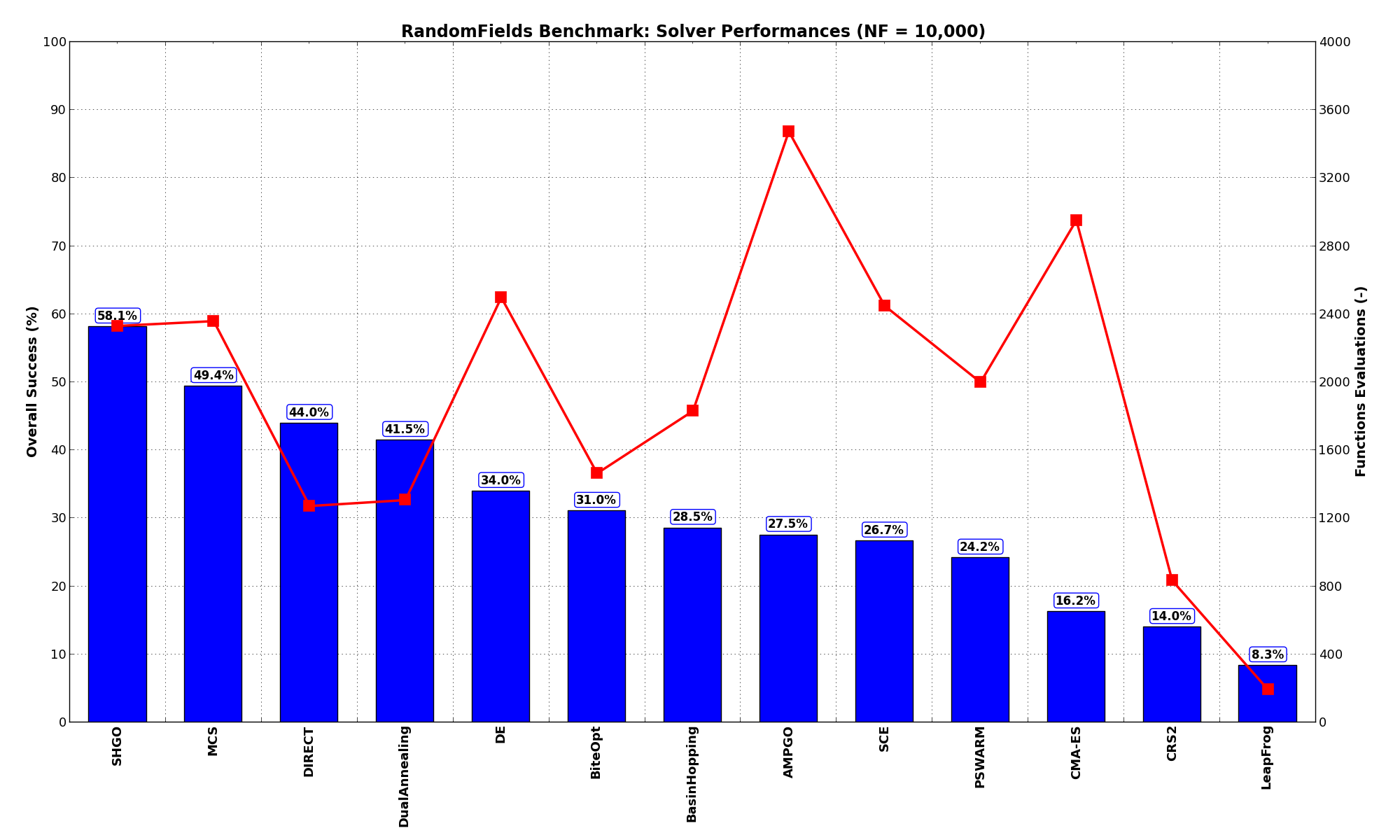Figure 10.3: Optimization algorithms performances on the RandomFields test suite at##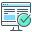Sensitivities on Functions Evaluations Budget¶

It is also interesting to analyze the success of an optimization algorithm based on the fraction (or percentage) of problems solved given a fixed number of allowed function evaluations, let’s say 100, 200, 300,... 2000, 5000, 10000.

In order to do that, we can present the results using two different types of visualizations. The first one is some sort of “small multiples” in which each solver gets an individual subplot showing the improvement in the number of solved problems as a function of the available number of function evaluations - on top of a background set of grey, semi-transparent lines showing all the other solvers performances.

This visual gives an indication of how good/bad is a solver compared to all the others as function of the budget available. Results are shown in Figure 10.4.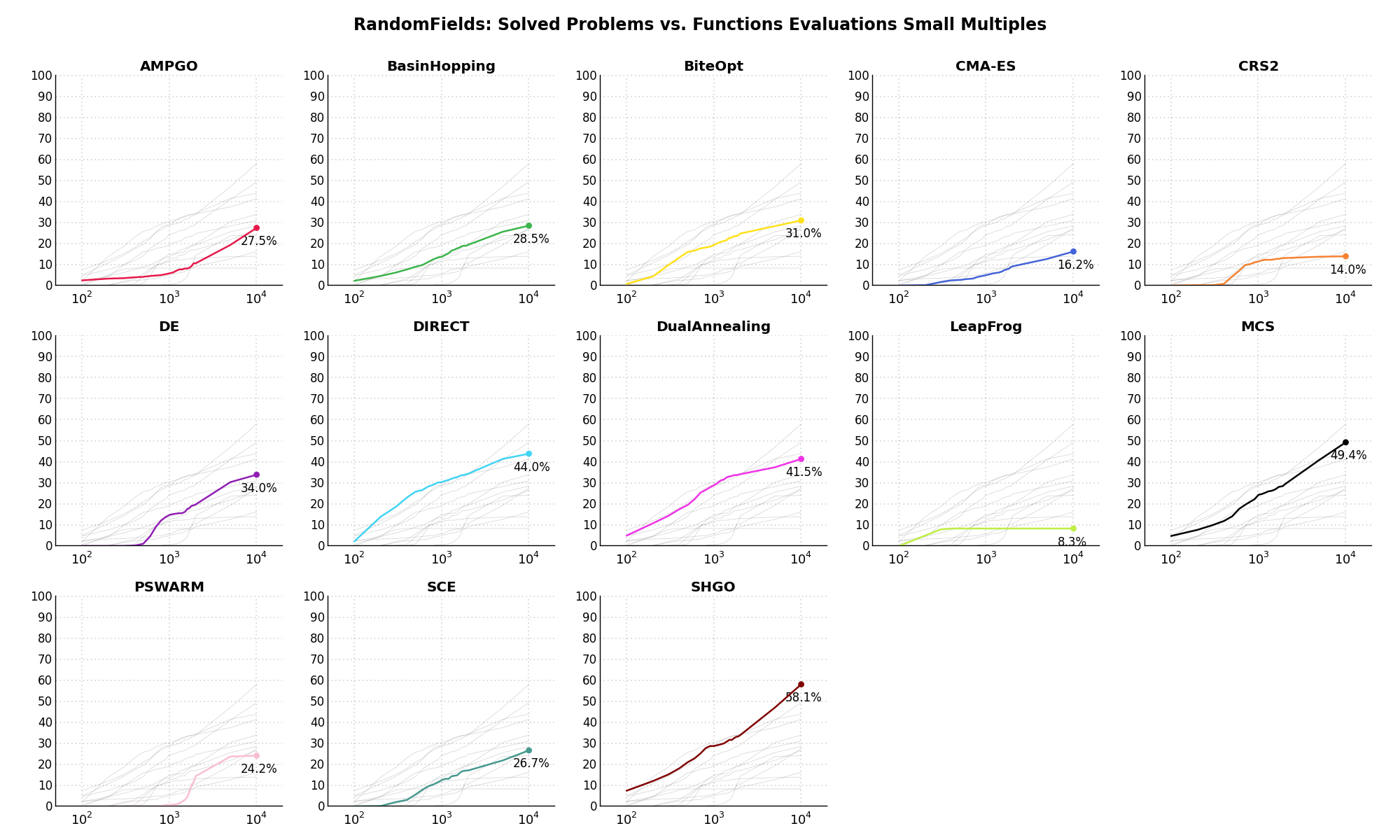Figure 10.4: Percentage of problems solved given a fixed number of function evaluations on the RandomFields test suite

The second type of visualization is sometimes referred as “Slopegraph” and there are many variants on the plot layout and appearance that we can implement. The version shown in Figure 10.5 aggregates all the solvers together, so it is easier to spot when a solver overtakes another or the overall performance of an algorithm while the available budget of function evaluations changes.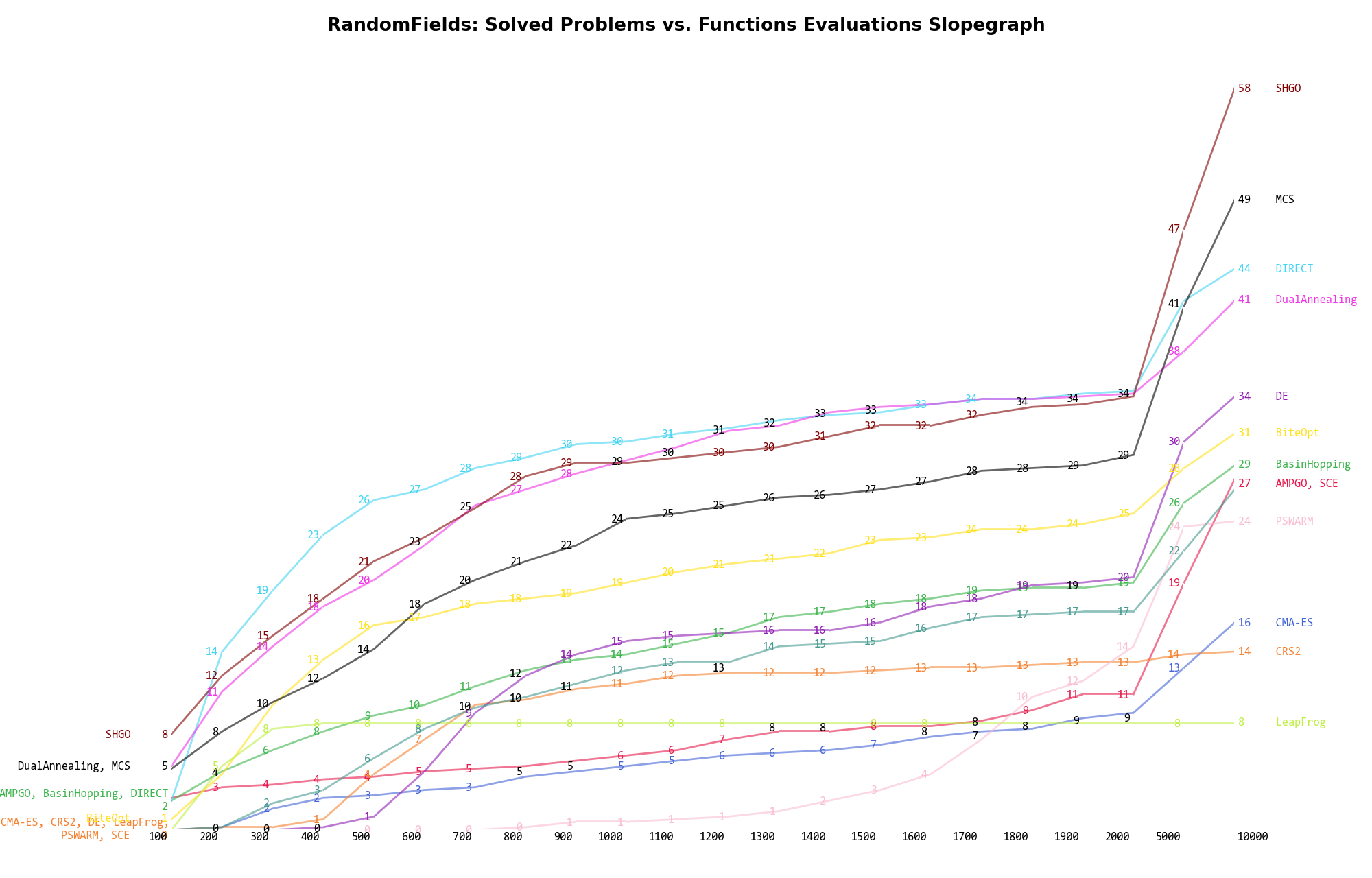Figure 10.5: Percentage of problems solved given a fixed number of function evaluations on the RandomFields test suite

A few obvious conclusions we can draw from these pictures are:

1. For this specific benchmark test suite, for lower function evaluations budgets, DIRECT, SHGO and DualAnnealing are the best choices.
2. The solvers mentioned above keep their lead even at higher function evaluations budgets, although MCS manages to climb back in second place if a large budget is given.

##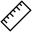Dimensionality Effects¶

Since I used the RandomFields test suite to generate test functions with dimensionality ranging from 2 to 5, it is interesting to take a look at the solvers performances as a function of the problem dimensionality. Of course, in general it is to be expected that for larger dimensions less problems are going to be solved - although it is not always necessarily so as it also depends on the function being generated. Results are shown in Table 10.3 .

Table 10.3: Dimensionality effects on the RandomFields benchmark suite at NF = 2,000
Solver N = 2 N = 3 N = 4 N = 5 Overall
AMPGO 27.5 8.3 3.3 3.3 10.6
BasinHopping 60.0 11.7 1.7 4.2 19.4
BiteOpt 60.0 20.0 5.8 13.3 24.8
CMA-ES 28.3 6.7 0.8 0.8 9.2
CRS2 40.0 7.5 2.5 2.5 13.1
DE 64.2 15.0 0.0 0.0 19.8
DIRECT 86.7 28.3 12.5 10.0 34.4
DualAnnealing 82.5 29.2 15.8 9.2 34.2
LeapFrog 21.7 6.7 1.7 3.3 8.3
MCS 83.3 17.5 11.7 5.0 29.4
PSWARM 51.7 1.7 0.8 3.3 14.4
SCE 63.3 4.2 0.8 0.0 17.1
SHGO 93.3 23.3 9.2 10.0 34.0

Figure 10.6 shows the same results in a visual way.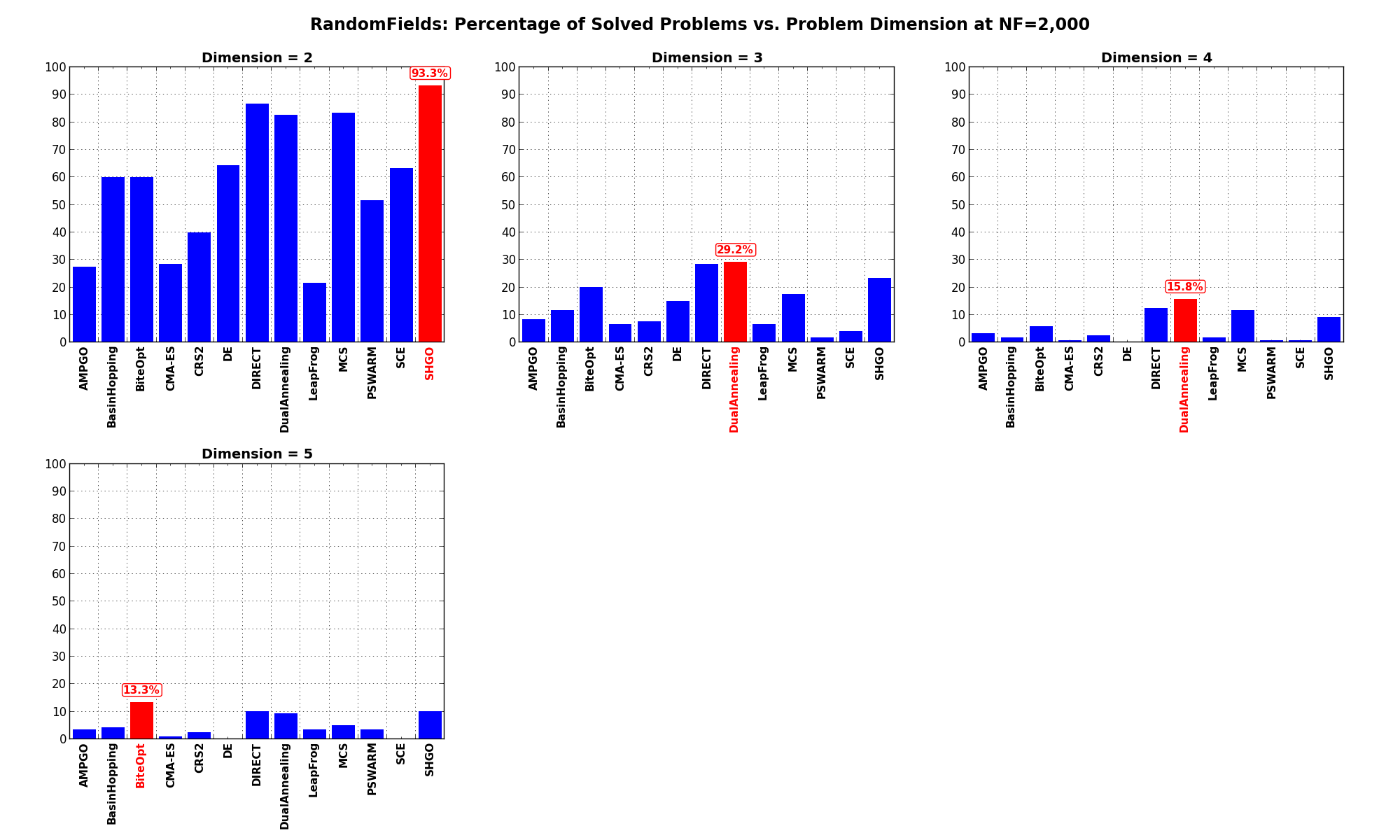Figure 10.6: Percentage of problems solved as a function of problem dimension for the RandomFields test suite atWhat we can infer from the table and the figure is that, for low dimensionality problems (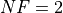), SHGO is able to solve most of the problems in this test suite, with DIRECT and MCS tightly following. By increasing the dimensionality the performances of all solvers degrade significantly, with DualAnnealing having a marginal advantage over other solvers.

Pushing the available budget to a very generousshows SHGO solving all problems, with MCS and DIRECT right behind for. For higher dimensionalities, again performance degradation is evident, although SHGO is the clear winner.

The results for the benchmarks atare displayed in Table 10.4 and Figure 10.7.

Table 10.4: Dimensionality effects on the RandomFields benchmark suite at NF = 10,000
Solver N = 2 N = 3 N = 4 N = 5 Overall
AMPGO 72.5 21.7 5.0 10.8 27.5
BasinHopping 73.3 24.2 6.7 10.0 28.5
BiteOpt 75.0 24.2 8.3 16.7 31.0
CMA-ES 43.3 11.7 5.8 4.2 16.2
CRS2 40.0 8.3 4.2 3.3 14.0
DE 64.2 40.0 25.8 5.8 34.0
DIRECT 95.8 42.5 17.5 20.0 44.0
DualAnnealing 85.8 39.2 24.2 16.7 41.5
LeapFrog 21.7 6.7 1.7 3.3 8.3
MCS 98.3 58.3 25.0 15.8 49.4
PSWARM 66.7 17.5 6.7 5.8 24.2
SCE 76.7 22.5 6.7 0.8 26.7
SHGO 100.0 66.7 23.3 42.5 58.1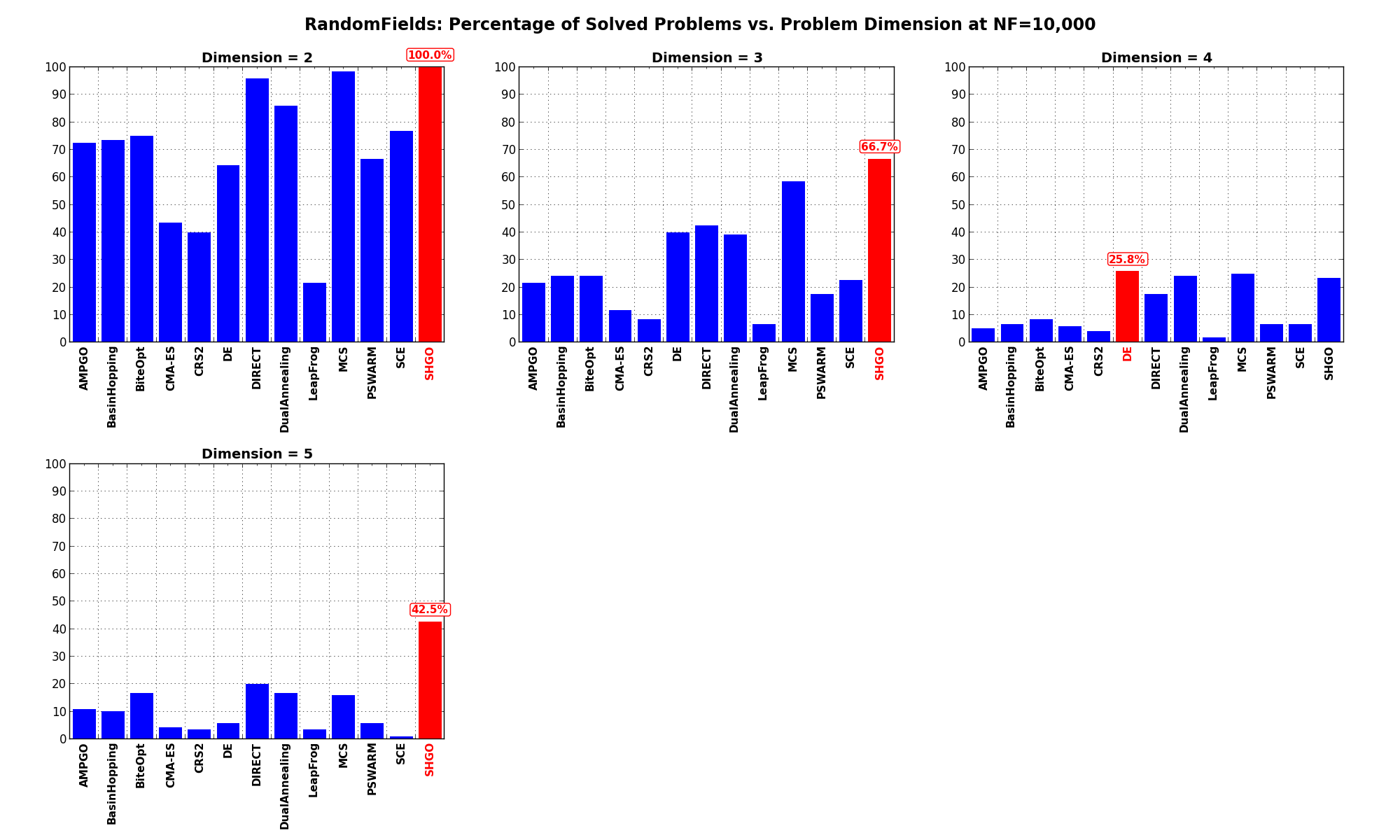Figure 10.7: Percentage of problems solved as a function of problem dimension for the RandomFields test suite at